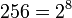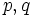GAP:SmallGroup

INTERNAL AD: Want to know more on this wiki about a group using its group ID? Type in SmallGroup(order,second part of ID) into the search box and get information on the wiki (not available for all groups). For instance, for the group with ID 34 among groups of order 120, type in SmallGroup(120,34).

Definition

Function type

SmallGroup is a GAP function that takes in two arguments, both of which are numbers. or alternatively a list of two numbers, and outputs a group.

Behavior

Consider the command SmallGroup(a,b) or SmallGroup([a,b]). Here is how this behaves:

Nature of input Output/error message Example inputs$a,b$ are positive integers, the order$a$ is in GAP's SmallGroup library, and there are at least$b$ groups of order$a$ The$b^{th}$ group among groups of order$a$ in GAP's SmallGroup library. SmallGroup(120,13)
SmallGroup(32,46)$a,b$ are positive integers, the order$a$ is in GAP's SmallGroup library, and there are fewer than$b$ groups of order$a$ error message specifying the number of groups of order$a$ SmallGroup(3,2)
SmallGroup(6,5)$a,b$ are positive integers, the groups of order$a$ are not in GAP's SmallGroup library error message stating that the library of groups of size$a$ is not available SmallGroup(1024,1)$a$ or$b$ are not positive integers, or other kinds of inputs are provided usage error SmallGroup(3,-1)

The following caveats should be noted about the manner in which the group is stored/processed:

• For a finite solvable group, the group is stored as a PcGroup: in other words, it is stored in terms of a polycyclic series for the group. Thus, if the group is solvable, the command SmallGroup returns a polycyclic series.
• For a finite group that is not solvable, the group is stored as a permutation group.

Available groups

The orders for which the SmallGroup function can be called are given below. See also AllSmallGroups.$2^n, 1 \le n \le 9$$2,4,8,16,32,64,128,256,512$ For orders$256 = 2^8$ and$512 = 2^9$, the default memory allocation provided by GAP is too small to manipulate the list of groups. A command line option -o 2G' is is usually enough for most simple manipulations involving the groups of orders 256 and 512. Also, to avoid wasteful printing of long output lists, use a double semicolon for output suppression.$p^n, 1 \le n \le 6$,$p$ prime (infinite list) For$n = 5$ and$n = 6$, the number of groups depends on$p$. As$p$ gets larger, additional memory allocation is needed.$p^7, p = 3,5,7,11$$3^7 = 2187, 5^7 = 78125, 7^7 = 823543, 11^7 = 19487171$ This is not available in the version of the library that came with GAP 4.4.12. The new version with these groups can be downloaded from here
cube-free number less than$50000 = 5 \cdot 10^4$ Too long to list
order$pq^n$,$p,q$ distinct primes, and$q^n$ divides one of these:$2^8,3^6, 5^5, 7^4$ (infinite list)
square-free number (infinite list)
order factorizes into at most 3 primes counting multiplicities (infinite list) Note here that "at most 3 primes" counts primes with multiplicity, so$p^3q^2r^4$ does not qualify.

The smallest orders for which the SmallGroups library does not have information are given in the table below:

Number Prime factorization
1024$\! 2^{10}$
2016$2^5 \cdot 3 \cdot 7$
2024$2^3 \cdot 11 \cdot 23$
2025$3^4 \cdot 5^2$
2040$2^3 \cdot 3 \cdot 5 \cdot 17$

Related functions

Aggregative functions

Function Description
SmallGroupsInformation Takes a single input$a$ and prints out verbal summary information on the groups of order$a$.
AllSmallGroups Returns, in list format, all the groups of a given order, say$a$. The$b^{th}$ member of the list is the same as the$b^{th}$ group of order$a$ invoked by the command SmallGroup(a,b);.
OneSmallGroup This returns just one group of a given order. It returns the first small group. Thus, the commands OneSmallGroup(a) and SmallGroup(a,1) have the same effect.
NumberSmallGroups This returns the number of small groups of a given order.

Reverse functions

• GAP:IdGroup: This takes a given group as input and outputs its group ID, i.e., an ordered pair of its order and the position it is in among the groups of that order. This is precisely the two-sided inverse of the SmallGroup function. Note that IdGroup is not available for some of the groups that have group IDs, specifically, groups of order 512, 1536, and those of order$p^5, p^6, p^7$ when the corresponding value is bigger than 2000 (with the exception of$5^5$, which is 3125).

Examples of usage

Single command examples

Here are some examples:

gap> SmallGroup(1,1);
<pc group of size 1 with 0 generators>
gap> IsTrivial(SmallGroup(1,1));
true
gap> SmallGroup(3,1);
<pc group of size 3 with 1 generators>


Below is an explanation:

Command GAP output/print Other GAP functions used Explanation
SmallGroup(1,1); <pc group of size 1 with 0 generators> -- The command gives the trivial group. The output by GAP for the first command is the output provided for any PcGroup: it gives the size, and the number of generators used for the polycyclic series. In this case, the trivial group has size one and the number of generators is zero.
IsTrivial(SmallGroup(1,1)); true IsTrivial This checks whether the group constructed as SmallGroup(1,1) is the trivial group. It is.
SmallGroup(3,1); <pc group of size 3 with 1 generators> -- This constructs the the cyclic group on three elements. Here, there is$1$ generator.

Error message examples

gap> H := SmallGroup(3,2);
Error, there is just 1 group of size 3 called from
SMALL_GROUP_FUNCS[inforec.func]( size, i, inforec ) called from
<function>( <arguments> ) called from read-eval-loop
you can 'quit;' to quit to outer loop, or
you can 'return;' to continue
brk> quit;
gap> SmallGroup(1024,1);
Error, the library of groups of size 1024 is not available called from
<function>( <arguments> ) called from read-eval-loop
you can 'quit;' to quit to outer loop, or
you can 'return;' to continue
Command Error message Explanation
H := SmallGroup(3,2); Error, there is just 1 group of size 3 ... The command tries to construct the second group of order three. But there is only one group of order three, and GAP returns the relevant error.
SmallGroup(1024,1); Error, the library of groups of size 1024 is not available ... The error message says that the order 1024 is not part of GAP's SmallGroup library.

Typical command sequences using SmallGroup

gap> G := SmallGroup(6,2);
<pc group of size 6 with 2 generators>
gap> G = CyclicGroup(6);
false
gap> IsomorphismGroups(G,CyclicGroup(6));
[ f1, f2 ] -> [ f1*f2, f2 ]
Command GAP output/print Other GAP functions used Explanation
G := SmallGroup(6,2); <pc group of size 6 with 2 generators> -- This constructs the cyclic group on six elements, and stores this group as$G$. Note that although this group has a generating set of size one, the polycyclic series has length two; so the number of generators used in this series is two.
G = CyclicGroup(6); false CyclicGroup This asks whether$G$ is equal, as the group is stored, to the cyclic group of order 6. The answer of false simply indicates that they are not equal in a literal sense, though as the next command shows, they are isomorphic groups.
IsomorphismGroups(G,CyclicGroup(6)); [ f1, f2 ] -> [ f1*f2, f2 ] CyclicGroup, IsomorphismGroups This tests whether the group$G$ is indeed isomorphic to the cyclic group of order six. It is, and the output specifies an isomorphism in terms of the way the groups are stored in GAP.

Here is another example:

gap> L := SmallGroup(60,5);
Group([ (1,2,3,4,5), (1,2,3) ])
gap> IsSimpleGroup(L);
true
gap> IsAlternatingGroup(L);
true

Below is an explanation:

Command GAP output/print Other GAP functions used Explanation
L := SmallGroup(60,5); Group([ (1,2,3,4,5), (1,2,3) ]) Constructs the group with GAP ID 5 among the groups of order 60. This is in fact isomorphic to alternating group:A5. Note that the group is stored using a permutation representation because it is not solvable.
IsSimpleGroup(L); true IsSimpleGroup Asks if the group is a simple group. It is.
IsAlternatingGroup(L); true IsAlternatingGroup Asks if the group is an alternating group. It is.
gap> M := SmallGroup(60,1);
<pc group of size 60 with 4 generators>
gap> IsCyclic(M);
false
gap> IsAbelian(M);
false
gap> IsSolvable(SmallGroup(60,1));
true
gap> StructureDescription(M);
"C5 x (C3 : C4)"`

Below is an explanation:

Command GAP output/print Other GAP functions used Explanation
M := SmallGroup(60,1); <pc group of size 60 with 4 generators> -- Constructs the group of GAP ID 1 among groups of order 60 and stores it as$M$. Note that it is stored as a PcGroup indicating that it is solvable.
IsCyclic(M); false IsCyclic Checks whether$M$ is a cyclic group. It is not.
IsAbelian(M); false IsAbelian Checks whether$M$ is an abelian group. It is not.
IsSolvable(SmallGroup(60,1)); true IsSolvable Checks whether$M$ (not written as$M$, but using the SmallGroup description) is solvable. It is solvable.
StructureDescription(M); "C5 x (C3 : C4)" StructureDescription Provides a (partial) description of the structure of$M$.